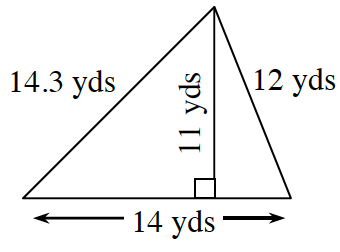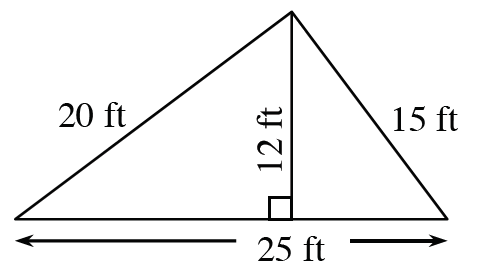### Home > CC3MN > Chapter 7 > Lesson 7.3.1 > Problem7-96

7-96.

Find the perimeter and area of each triangle below.

1.The perimeter is the sum of all the sides of the shape.

$14.3+12+14=$ perimeter

Remember to include units.

The area of a triangle can be found using the formula:

$\text{Area} = \frac{1}{2}\text{(base)} \text{(height)}$

Remember to include units.

1.See part (a).